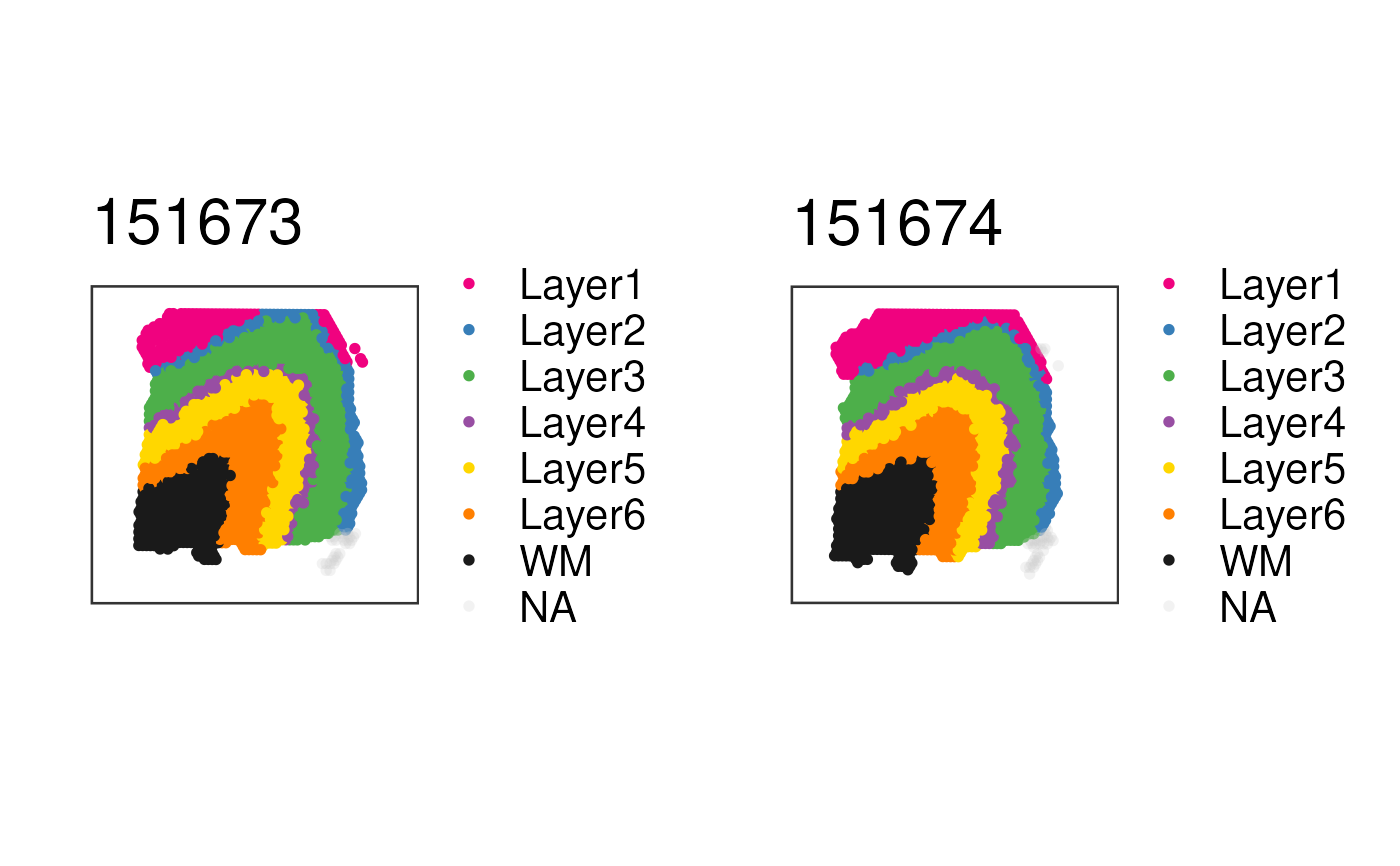This function visualizes the clusters for a set of samples at the spot-level using (by default) the histology information on the background. To visualize gene-level (or any continuous variable) use vis_grid_gene().

vis_grid_clus(
spe,
clustervar,
pdf_file,
sort_clust = TRUE,
colors = NULL,
return_plots = FALSE,
spatial = TRUE,
height = 24,
width = 36,
image_id = "lowres",
alpha = NA,
sample_order = unique(spe$sample_id), point_size = 2, auto_crop = TRUE, ... ) ## Arguments spe Defaults to the output of fetch_data(type = 'spe'). This is a SpatialExperiment-class object with the spot-level Visium data and information required for visualizing the histology. See fetch_data() for more details. clustervar A character(1) with the name of the colData(spe) column that has the cluster values. pdf_file A character(1) specifying the path for the resulting PDF. sort_clust A logical(1) indicating whether you want to sort the clusters by frequency using sort_clusters(). colors A vector of colors to use for visualizing the clusters from clustervar. If the vector has names, then those should match the values of clustervar. return_plots A logical(1) indicating whether to print the plots to a PDF or to return the list of plots that you can then print using plot_grid. spatial A logical(1) indicating whether to include the histology layer from geom_spatial(). If you plan to use ggplotly() then it's best to set this to FALSE. height A numeric(1) passed to pdf. width A numeric(1) passed to pdf. image_id A character(1) with the name of the image ID you want to use in the background. alpha A numeric(1) in the [0, 1] range that specifies the transparency level of the data on the spots. sample_order A character() with the names of the samples to use and their order. point_size A numeric(1) specifying the size of the points. Defaults to 1.25. Some colors look better if you use 2 for instance. auto_crop A logical(1) indicating whether to automatically crop the image / plotting area, which is useful if the Visium capture area is not centered on the image and if the image is not a square. ... Passed to paste0() for making the title of the plot following the sampleid. ## Value A list of ggplot2 objects. ## Details This function prepares the data and then loops through vis_clus() for computing the list of ggplot2 objects. ## See also Other Spatial cluster visualization functions: frame_limits(), vis_clus_p(), vis_clus() ## Examples  if (enough_ram()) { ## Obtain the necessary data if (!exists("spe")) spe <- fetch_data("spe") ## Subset to two samples of interest and obtain the plot list p_list <- vis_grid_clus( spe[, spe$sample_id %in% c("151673", "151674")],
"layer_guess_reordered",
spatial = FALSE,
return_plots = TRUE,
sort_clust = FALSE,
colors = libd_layer_colors
)

## Visualize the spatial adjacent replicates for position = 0 micro meters
## for subject 3
cowplot::plot_grid(plotlist = p_list, ncol = 2)
}